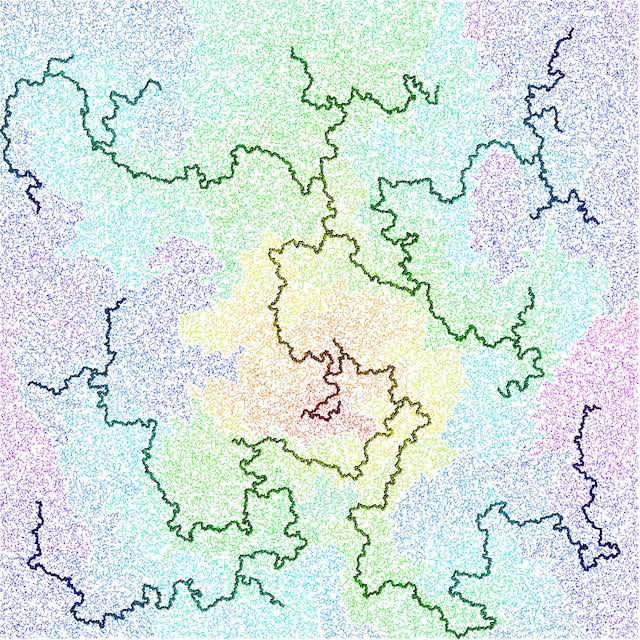Home  /  Probability and statistics of discrete structures

# Program

Probability and Statistics of Discrete Structures January 21, 2025 to May 16, 2025
Organizers Louigi Addario-Berry (McGill University), Christina Goldschmidt (University of Oxford), Po-Ling Loh (University of Cambridge), Gabor Lugosi (ICREA), Dana Randall (Georgia Institute of Technology), LEAD Remco van der Hofstad (Technische Universiteit Eindhoven)
DescriptionThe minimum spanning tree of 100,000 uniformly random points. Colors encode graph distance from the root, which is red. Black points are those whose removal would disconnect at least 5% of the points from the rest.
Random graphs and related random discrete structures lie at the forefront of applied probability and statistics, and are core topics across a wide range of scientific disciplines where mathematical ideas are used to model and understand real-world networks. At the same time, random graphs pose challenging mathematical and algorithmic problems that have attracted attention from probabilists and combinatorialists since at least 1960, following the pioneering work of Erdős and Renyi. Around the turn of the millennium, as very large data sets became available, several applied disciplines started to realize that many real-world networks, even though they are from various origins, share fascinating features. In particular, many such networks are small worlds, meaning that graph distances in them are typically quite small, and they are scale-free, in the sense that the number of connections made by their elements is extremely heterogeneous. This program is devoted to the study of the probabilistic and statistical properties of such networks. Central tools include graphon theory for dense graphs, local weak convergence for sparse graphs, and scaling limits for the critical behavior of graphs or stochastic processes on them. The program is aimed at pure and applied mathematicians interested in network problems.
Keywords and Mathematics Subject Classification (MSC)
Tags/Keywords
• Structure of/optimization on random graphs

• statistics of networks

• counting and sampling discrete structures

• estimation of network parameters

• complexity vs. statistical accuracy

• community detection

• combinatorial statistics

• random processes on graphs

• randomized algorithms on random graphs

• probabilistic analysis of network algorithms

Primary Mathematics Subject Classification
Secondary Mathematics Subject Classification
Logistics Program Logistics can be viewed by Members. If you are a program member then Login Here.
Programmatic Workshops
 January 23, 2025 - January 24, 2025 Connections Workshop: Probability and Statistics of Discrete Structures January 27, 2025 - January 31, 2025 Introductory Workshop: Probability and Statistics of Discrete Structures April 21, 2025 - April 25, 2025 Detection, Estimation, and Reconstruction in Networks